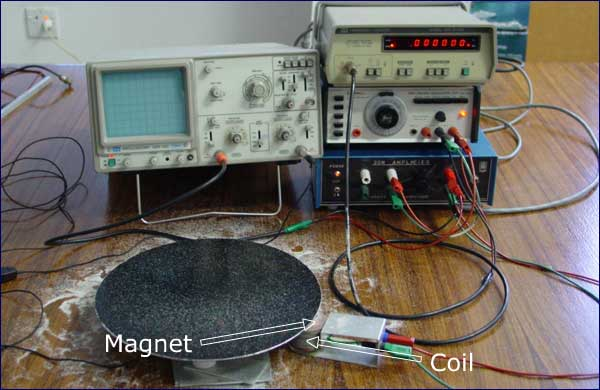# Simple Harmonic Motion -- background material

Simple harmonic motion (SHM) -- some examples. The first animation is a cartoon describing aspects of one state of the quantum mechanical wave function of a 'an electron in a box' -- an electron in a two dimensional potential well with infinite walls. For a small amplitude oscillation, a pendulum is a simple hamonic oscillator. Loudspeakers can reproduce single SHM, which is not very interesting, or combinations of of SHM, from which we can make any sound.

### Simple harmonic motion as a projection of uniform circular motion

Here we see that the vertical component of uniform circular motion is SHM. For uniform circular motion with θ = ωt and radius r, the vertical component is

y  =  r sin θ  =  r sin ωt

### Displacement, velocity and acceleration in simple harmonic motion

In the animation above, the purple curve is the displacement y as a funciton of t, ie y(t). The red tangent shows its slope, which is dy/dt, the velocity vy, which is shown in the next graph. On that graph, the blue tangent shows the slope dvy/dt, which is the vertical acceleration ay., which is shown in the third graph. (Revise calculus)

Let's look at how vy and ay depend on ω. For SHM with amplitude A, let's write

y  =  A sin ωt       so
vy  =  dy/dt  =  Aω cos ωt       and
ay  =  dvy/dt  =  − Aω2 cos ωt .
These are plotted below, for two different values of ω: in the faint version ω is doubled.Displacement, velocity and acceleration graphs for SHM

If we double ω we double the number of cycles per unit time, so we cover twice as much distance in the same time -- vy is doubled. Similarly, the acceleration goes through twice as many cycles in the same time. But more than that, the acceleration involves velocities that are twice as large, so ay is four times greater.

### Chladni patterns as examples of simple harmonic motionExperimental set up for Chladni patterns

In Chladni patterns, points in an object undergo SHM with varying amplitudes. Small particles (sand in this case) collect at the nodes, ie along lines where the amplitude of SHM is small.

The same object usually has more than one resonance.

### A spectrum represents the sum of simple harmonic oscillationsThis work is licensed under a Creative Commons License.